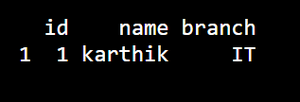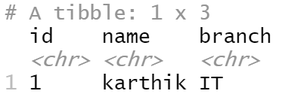# Convert Named Vector to DataFrame in R

In this article, we will see how to convert the named vector to Dataframe in the R Programming Language.

Method 1:

Generally while converting a named vector to a dataframe we may face a problem. That is, names of vectors may get converted into row names, and data may be converted into a single column. So we need to convert the vector into a list then convert the list into a dataframe.

First, we will convert the vector into a list using as.list( ) method and passed it to data.frame( ) method in order to convert the vector into dataframe.

Example:

## R

 `vector1 = ``c``(1, ``"karthik"``, ``"IT"``) ` `names``(vector1) = ``c``(``"id"``, ``"name"``, ``"branch"``) ` ` `  `df = ``data.frame``(``as.list``(vector1)) ` `print``(df)`

Output :Method 2: Using tibble library.

In tibble library there is a method called as_tibble( ) function. In order to use as_tibble( ) we need to install tibble library. To install package we can use install.packages( ) function by passing package name as parameter.

syntax : variable = as_tibble (as.list(vector))

Example:

## R

 `library``(tibble) ` `vec1 = ``c``(``"1"``, ``"karthik"``, ``"IT"``) ` `names``(vec1) = ``c``(``"id"``, ``"name"``, ``"branch"``) ` ` `  `df=``as_tibble``(``as.list``(vec1)) ` `print``(df) `

Output:Whether you're preparing for your first job interview or aiming to upskill in this ever-evolving tech landscape, GeeksforGeeks Courses are your key to success. We provide top-quality content at affordable prices, all geared towards accelerating your growth in a time-bound manner. Join the millions we've already empowered, and we're here to do the same for you. Don't miss out - check it out now!

Previous
Next